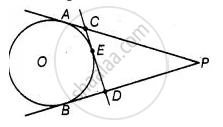# From an external point P, two tangents, PA and PB are drawn to a circle with centre O. At one point E on the circle tangent is drawn which intersects PA and PB at C and D, respectively - Mathematics

Diagram
Sum

From an external point P, two tangents, PA and PB are drawn to a circle with centre O. At one point E on the circle tangent is drawn which intersects PA and PB at C and D, respectively. If PA = 10 cm, find the the perimeter of the triangle PCD.

#### Solution

Two tangents PA and PB are drawn to a circle with centre 0 from an external point PPerimeter of ΔPCD = PC + CD + PD

= PC + CE + ED + PD

= PC + CA + DB + PD

= PA + PB

= 2PA

= 2(10)

= 20 cm  .......[∵ CE = CA, DE = DB, PA = PB tangents from internal point to a circle are equal]

Concept: Concept of Circle - Centre, Radius, Diameter, Arc, Sector, Chord, Segment, Semicircle, Circumference, Interior and Exterior, Concentric Circles
Is there an error in this question or solution?

#### APPEARS IN

NCERT Mathematics Exemplar Class 10
Chapter 9 Circles
Exercise 9.4 | Q 3 | Page 110
Share